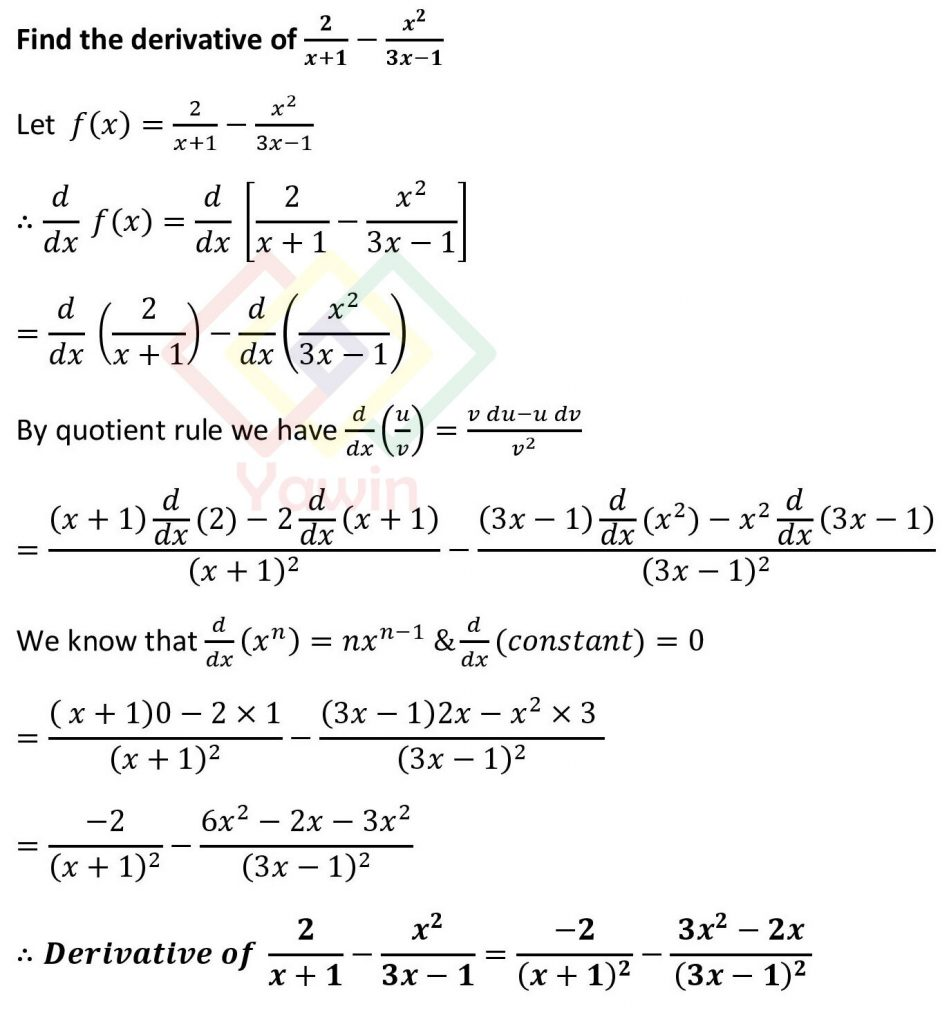Find the derivative of (2/(x+1))-(x^2/(3x-1))

## Problem## Question

Find the derivative of (2/(x+1))-(x^2/(3x-1))